# NTNUJAVA Virtual Physics LaboratoryEnjoy the fun of physics with simulations! Backup site http://enjoy.phy.ntnu.edu.tw/ntnujava/

## Easy Java Simulations (2001- ) => Collaborative Community of EJS => Topic started by: lookang on May 20, 2011, 10:44:32 pm

Title: Ejs Open Source Newton's Mountain Projectile Orbits Model java applet
Post by: lookang on May 20, 2011, 10:44:32 pm
Ejs Open Source Newton Mountain Model java applet by Todd Timberlake remixed by lookang
Ejs Open Source Newton's Mountain Projectile Orbits Model java applet
reference:
http://www.phy.ntnu.edu.tw/ntnujava/index.php?topic=398.msg7167#msg7167

Title: Re: Ejs Open Source Newton Mountain Model java applet
Post by: lookang on May 20, 2011, 10:48:30 pm
changes:
made sliders and fields conform to my design preference
redo for SI units for closer to sg syllabus instead of arbitrary units originally made
22May2011
add panel of x,y,vx,vy,ax,ay for calculating values to coincident with Earth data
add trace instead of the older trail that can display older data
rotate newton's diagram by 5 degree to coincident with x=0, instead of the older 0.1*R
change to acceleration instead of force
24 May 2011
add multiple representation of versus time and distance from center of earth
add menu for circular motion R,2R,3R and escape velocity at theta 0,45,90
26 May 2011
minor changes on menu list name R_earth instead of 6.38E06 as suggested by leongster
add check box on the bottom panel for quick access as suggested by leongster.
07 june2011
added reference frame space and earth for earth to rotate if frame is space
added a few geostationary orbits and non-geostationary
remove newton mountain and replace with a rectangle

to do
newton mountain gif to make background transparent

challenge:
some problem detecting the boundary of earth solved

double hitEarth (double x, double y) {
if (!pass) {
return x*x+y*y-R*R;}
else {
return 0;}
}

Title: Re: Ejs Open Source Newton's Mountain Projectile Orbits Model java applet
Post by: lookang on May 23, 2011, 09:26:45 pm
question.
what is the velocity of the projectile if it is launch horizontally at the surface of the Earth?

hint: use circular motion equation to find the v!

enjoy!

7920.05 m/s

try it with the this customized with Earth data simulation :)

Title: Re: Ejs Open Source Newton's Mountain Projectile Orbits Model java applet
Post by: lookang on October 08, 2012, 05:32:34 pm

Orbits round the Earth

An object projected horizontally near the Earth’s surface follows a parabolic trajectory. As the velocity of projection increases, there will be an occasion in which the trajectory follows the curvature of the Earth’s surface. If air resistance is negligible, the object will orbit round the Earth and will never meet the Earth’s surface.speed of cannonball at 0 km/hr launched horizontally from newton's very tall mountain, resulting in trajectory that hits Earth surface.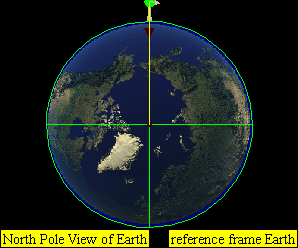speed of cannonball at 1 000 km/hr launched horizontally from newton's very tall mountain, resulting in trajectory that hits Earth surface.speed of cannonball at 2 000 km/hr launched horizontally from newton's very tall mountain, resulting in trajectory that hits Earth surface.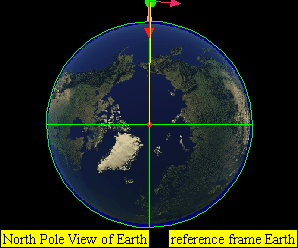speed of cannonball at 3 000 km/hr launched horizontally from newton's very tall mountain, resulting in trajectory that hits Earth surface.speed of cannonball at 4 000 km/hr launched horizontally from newton's very tall mountain, resulting in trajectory that hits Earth surface.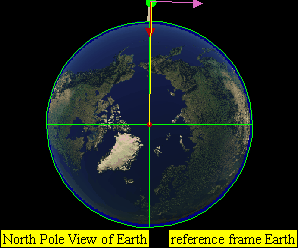speed of cannonball at 5 000 km/hr launched horizontally from newton's very tall mountain, resulting in trajectory that hits Earth surface.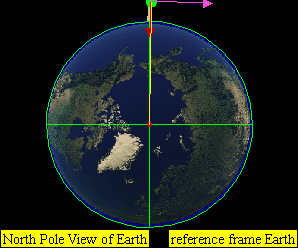speed of cannonball at 6 000 km/hr launched horizontally from newton's very tall mountain, resulting in trajectory that hits Earth surface.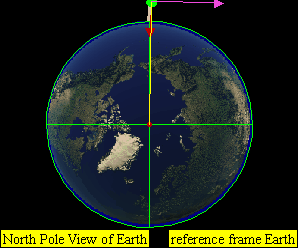speed of cannonball at 7 000 km/hr launched horizontally from newton's very tall mountain, resulting in trajectory that does NOT hit the Earth surface.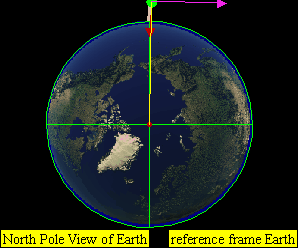speed of cannonball at 7 300 km/hr launched horizontally from newton's very tall mountain, resulting in trajectory orbit that does NOT hit the Earth surface that is a approximately a circular orbit.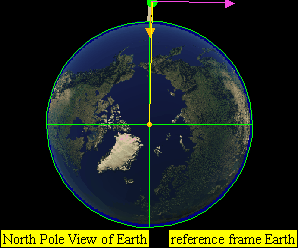speed of cannonball at 8 000 km/hr launched horizontally from newton's very tall mountain, resulting in trajectory orbit that does NOT hit the Earth surface.speed of cannonball at 9 000 km/hr launched horizontally from newton's very tall mountain, resulting in trajectory orbit that does NOT hit the Earth surface.speed of cannonball at 11 200 km/hr launched horizontally from newton's very tall mountain, resulting in trajectory that does NOT hit the Earth surface and escape from the Earth's gravitational pullplot of kinetic energy$E_{K}$, gravitational potential energy,$E_{P}$ and total Energy$E$versus distance away from centre of earth, r at R= Re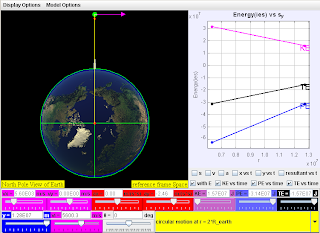plot of kinetic energy$E_{K}$, gravitational potential energy,$E_{P}$ and total Energy$E$versus distance away from centre of earth, r at R= Re, R= 2*Re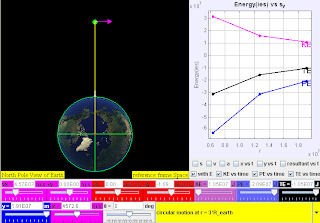plot of kinetic energy$E_{K}$, gravitational potential energy,$E_{P}$ and total Energy$E$versus distance away from centre of earth, r at R= Re, R= 2*Re, R=3*Replot of kinetic energy$E_{K}$, gravitational potential energy,$E_{P}$ and total Energy$E$versus distance away from centre of earth, r at R= Re, R= 2*Re, R=3*Re and lastly R = geostationary radius

Title: Re: Ejs Open Source Newton's Mountain Projectile Orbits Model java applet
Post by: lookang on October 09, 2012, 03:19:54 pm
Question01
An
earth satellite of mass 200 kg lost energy slowly through atmospheric
resistance and fell from an orbit of radius 8.0 x 106 m to 7.8 x 106 m.
Calculate the changes in the potential, kinetic and total energies of
the satellite during this transition period.

Answers: ( -2.57E8 J, 1.28E8 J, -1.28E8 J ) where E denotes x10

Solution:

a)b)

Assuming the orbits are in circular motion as it decays fromtoequationcan be assumed to be valid to approximate this motion

Thus,c)

sincecheck:

This answercan also be verified from the formula relationship on page Newton's Mountain Projectile Orbits which is not intended to be memorized.

Using the simulation:using R = 8.0X10^6 , v = 7072.8 m/s for circular motion orbit, you can get for satellite m = 1kg, KE = 2.501X10^7 J, PE = -5.002X10^7 J and TE = -2.501X10^7 Jusing R = 7.8X10^6 , v = 7162.9 m/s for circular motion orbit, you can get for satellite m = 1kg, KE = 2.565X10^7 J, PE = -5.131X10^7 J and TE = -2.565X10^7 J

Thus,

ΔKE = 200(2.565X10^7 - 2.501X10^7) = 1.28X10^8 J

ΔPE = 200(-5.131X10^7 - (-5.002X10^7)) = -2.58X10^8 J

ΔTE = 1.28X10^8 + (-2.58X10^8) = 1.30X10^8 J

where the slight difference is due to carry over error from the significant figures of the computer model.

reference:

http://commons.wikimedia.org/wiki/File:R_%3D_geo_Re_2012-10-08_1809.png#.7B.7Bint:filedesc.7D.7D

Title: Re: Ejs Open Source Newton's Mountain Projectile Orbits Model java applet
Post by: lookang on June 17, 2013, 07:34:00 am
taking the codes from fu-kwun hwang model here http://www.phy.ntnu.edu.tw/ntnujava/index.php?topic=2083.0
i added the ability to fire and circular from this model space shuttle motion with impulse and added into the model above Ejs Open Source Newton's Mountain Projectile Orbits Model java applet http://www.phy.ntnu.edu.tw/ntnujava/index.php?topic=2204.0 !

enjoy!

http://weelookang.blogspot.sg/2011/05/ejs-open-source-newtons-mountain.html
added ability to fire to add velocity to a achieve eclipse orbit and select circular to achieve circular orbit by applying impulse in directions to get velocity at the correct speed and direction, perpendicular to radius.  this feature helps in the learning of energies change as satellite move from one circular orbit to another circular as requested by soo kok.
https://dl.dropboxusercontent.com/u/44365627/lookangEJSworkspace/export/ejs_NewtonsMountainwee06.jar
author: timberlake, lookang and  fu-kwun hwang

many thanks to prof fu-kwun hwang and his physics validated codes to help ordinary teachers make wonderful models for enhanced teaching and learning for the benefit of all humankind!